# Lecturers slides http www comp nus edu sgcs

• Slides: 16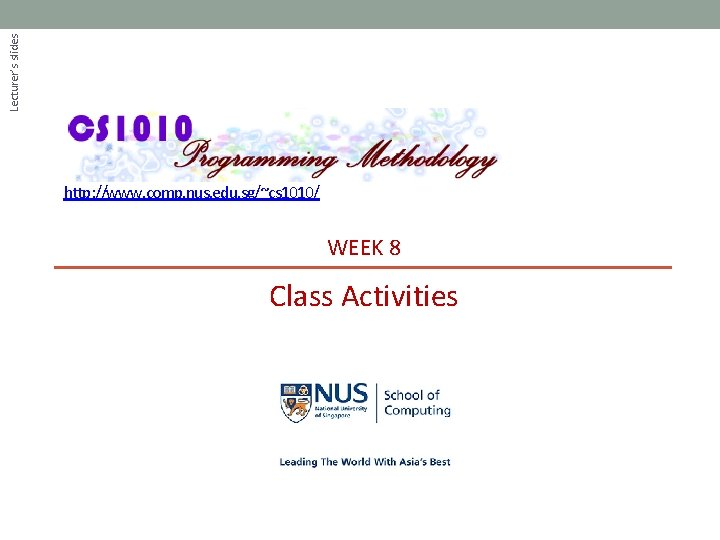Lecturer’s slides http: //www. comp. nus. edu. sg/~cs 1010/ WEEK 8 Class Activities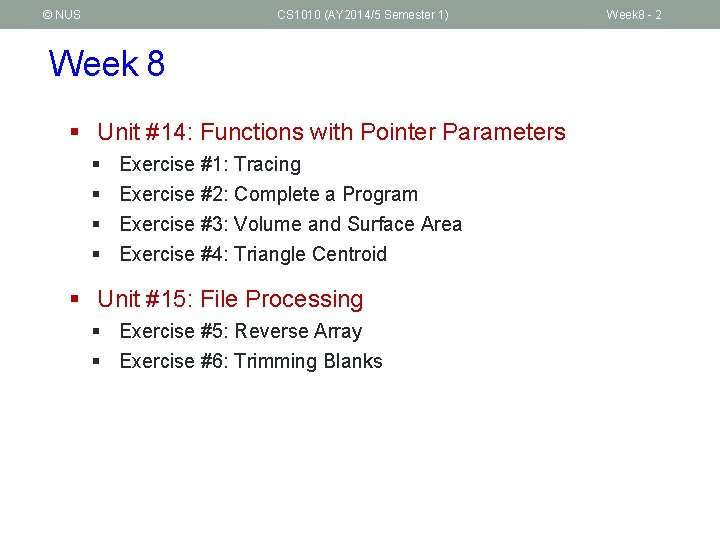© NUS CS 1010 (AY 2014/5 Semester 1) Week 8 § Unit #14: Functions with Pointer Parameters § § Exercise #1: Tracing Exercise #2: Complete a Program Exercise #3: Volume and Surface Area Exercise #4: Triangle Centroid § Unit #15: File Processing § Exercise #5: Reverse Array § Exercise #6: Trimming Blanks Week 8 - 2© NUS CS 1010 (AY 2014/5 Semester 1) Week 8 - 3 Week 8 Programs § Download the programs from this web page § http: //www. comp. nus. edu. sg/~cs 1010/lect/prog/2014/week 8_for_students § The files are: § § § array. in trimblanks. in Week 8_Max. Ave_Incomplete. c Week 8_Reverse. Array. c Week 8_Trace. c Week 8_Trim. Blanks. c § You may also copy the above files directly into your sunfire account using the following UNIX command, where xxx is the name of one of the above files: cp ~cs 1010/public_html/lect/prog/2014/week 8_for_students/xxx.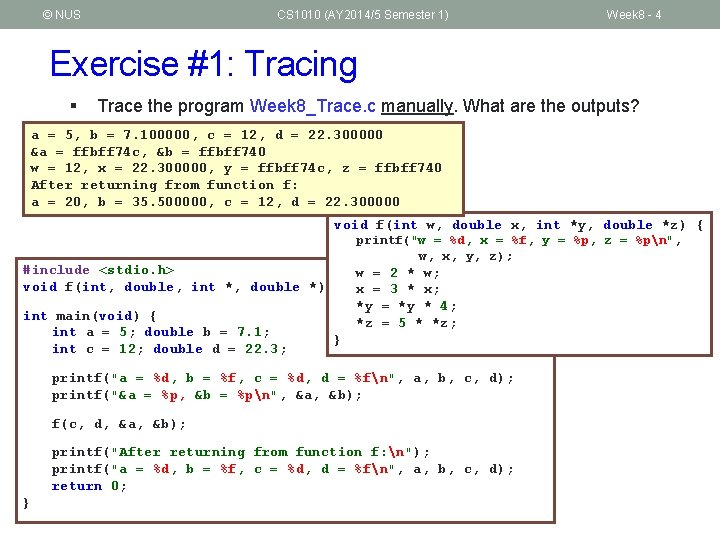© NUS CS 1010 (AY 2014/5 Semester 1) Week 8 - 4 Exercise #1: Tracing § Trace the program Week 8_Trace. c manually. What are the outputs? a = 5, b = 7. 100000, c = 12, d = 22. 300000 &a = ffbff 74 c, &b = ffbff 740 w = 12, x = 22. 300000, y = ffbff 74 c, z = ffbff 740 After returning from function f: a = 20, b = 35. 500000, c = 12, d = 22. 300000 void f(int w, double x, int *y, double *z) { printf("w = %d, x = %f, y = %p, z = %pn", w, x, y, z); #include <stdio. h> void f(int, double, int *, double *); int main(void) { int a = 5; double b = 7. 1; int c = 12; double d = 22. 3; w = 2 * w; x = 3 * x; *y = *y * 4; *z = 5 * *z; } printf("a = %d, b = %f, c = %d, d = %fn", a, b, c, d); printf("&a = %p, &b = %pn", &a, &b); f(c, d, &a, &b); printf("After returning from function f: n"); printf("a = %d, b = %f, c = %d, d = %fn", a, b, c, d); return 0; }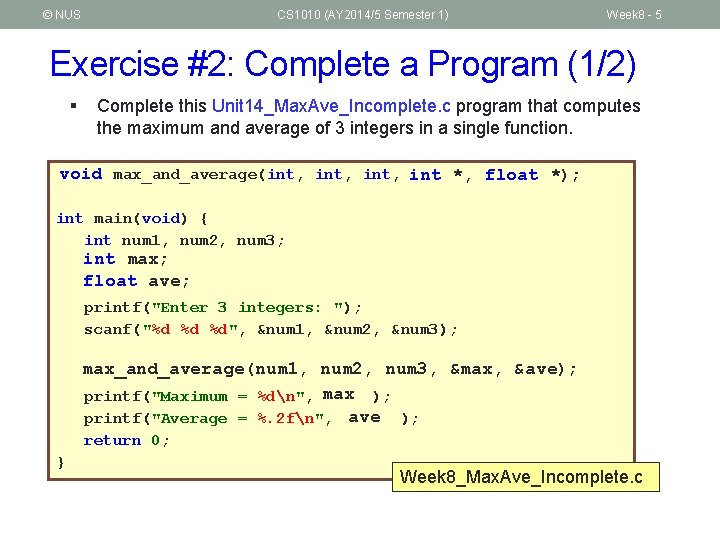© NUS CS 1010 (AY 2014/5 Semester 1) Week 8 - 5 Exercise #2: Complete a Program (1/2) § Complete this Unit 14_Max. Ave_Incomplete. c program that computes the maximum and average of 3 integers in a single function. void max_and_average(int, int *, float *); int main(void) { int num 1, num 2, num 3; int max; float ave; printf("Enter 3 integers: "); scanf("%d %d %d", &num 1, &num 2, &num 3); max_and_average(num 1, num 2, num 3, &max, &ave); printf("Maximum = %dn", max ); printf("Average = %. 2 fn", ave ); return 0; } Week 8_Max. Ave_Incomplete. c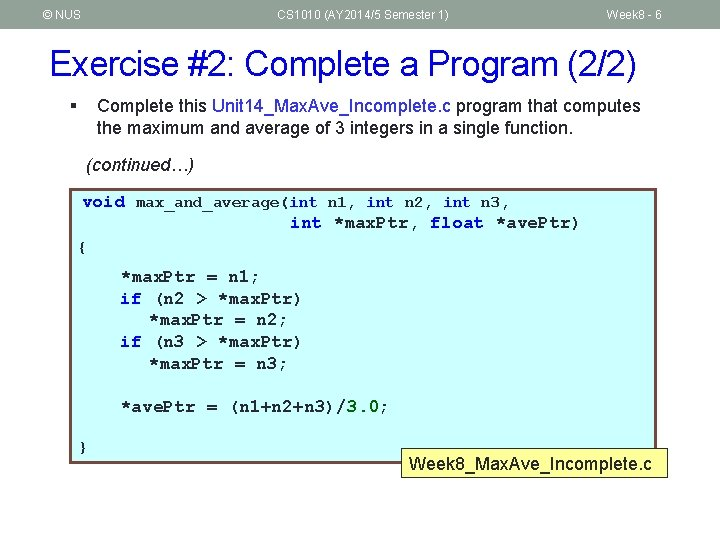© NUS CS 1010 (AY 2014/5 Semester 1) Week 8 - 6 Exercise #2: Complete a Program (2/2) § Complete this Unit 14_Max. Ave_Incomplete. c program that computes the maximum and average of 3 integers in a single function. (continued…) void max_and_average(int n 1, int n 2, int n 3, int *max. Ptr, float *ave. Ptr) { *max. Ptr = n 1; if (n 2 > *max. Ptr) *max. Ptr = n 2; if (n 3 > *max. Ptr) *max. Ptr = n 3; *ave. Ptr = (n 1+n 2+n 3)/3. 0; } Week 8_Max. Ave_Incomplete. c© NUS CS 1010 (AY 2014/5 Semester 1) Week 8 - 7 Exercise #3: Volume, Surface Area (1/2) h dt § Week 8_Cuboid_v 1. c: Include 2 functions volume(…) and surface_area(…) to compute the volume and surface area of the cuboid separately. § Week 8_Cuboid_v 2. c: Include a single function volume_and_surface_area(…) to compute both the volume and surface area of the cuboid. § There should be no printf() statement in your functions (apart from the main() function). length wi § You are to write 2 versions and compare them: depth § Write a program to read the length, width and depth (all integers) of a cuboid and compute (1) its volume, and (2) its surface area.© NUS CS 1010 (AY 2014/5 Semester 1) Week 8 - 8 Exercise #3: Volume, Surface Area (2/2) § Sample runs Enter length, width and depth: 6 3 10 Volume = 180 Surface area = 216 Enter length, width and depth: 15 14 12 Volume = 2520 Surface area = 1116© NUS CS 1010 (AY 2014/5 Semester 1) Week 8 - 9 Exercise #4: Triangle Centroid (1/2) § In a triangle, a median is a line that connects a vertex to the midpoint of its opposite side. (eg: blue dotted lines) § The intersection of the 3 medians is called the centroid. (eg: point G) P (x 1, y 1) G Q (x 2, y 2) R (x 3, y 3) § Write a program Week 8_Centroid. c to read in the coordinates (of type float) of 3 vertices of a triangle and compute the coordinates of its centroid. § Your program should have a function centroid(…). § There should be no printf() statement in this centroid() function. § If you can’t complete in class, continue to do it at home. § This exercise is mounted on Code. Crunch.© NUS CS 1010 (AY 2014/5 Semester 1) Exercise #4: Triangle Centroid (2/2) § Sample runs Coordinates of of 1 st vertex: 0 0 2 nd vertex: 0 1 3 rd vertex: 1 1 centroid = (0. 33, 0. 67) Coordinates of of 1 st vertex: 4. 8 12. 7 2 nd vertex: -12. 3 8. 2 3 rd vertex: -5. 6 15. 3 centroid = (-4. 37, 12. 07) Week 8 - 10© NUS CS 1010 (AY 2014/5 Semester 1) Week 8 - 11 Exercise #5: Reverse Array (1/3) § Given the program Week 8_Reverse. Array. c to read values into an integer array, reverse the array, and print the array after reversal. § Modify the program such that it reads from a text file “array. in” and writes to a text file “array. out”© NUS CS 1010 (AY 2014/5 Semester 1) Week 8 - 12 Exercise #5: Reverse Array (2/3) #include <stdio. h> #define MAX_SIZE 10 Week 8_Reverse. Array. c int scan. Array(int []); void print. Array(int [], int); void reverse. Array(int [], int); int main(void) { int array[MAX_SIZE], size; size = scan. Array(array); // Reverse the array void reverse. Array(int arr[], int size) { int i, temp; reverse. Array(array, size); for (i=0; i<size/2; i++) { temp = arr[i]; arr[i] = arr[size-i-1]; arr[size-i-1] = temp; } printf("After reversing: "); print. Array(array, size); return 0; } }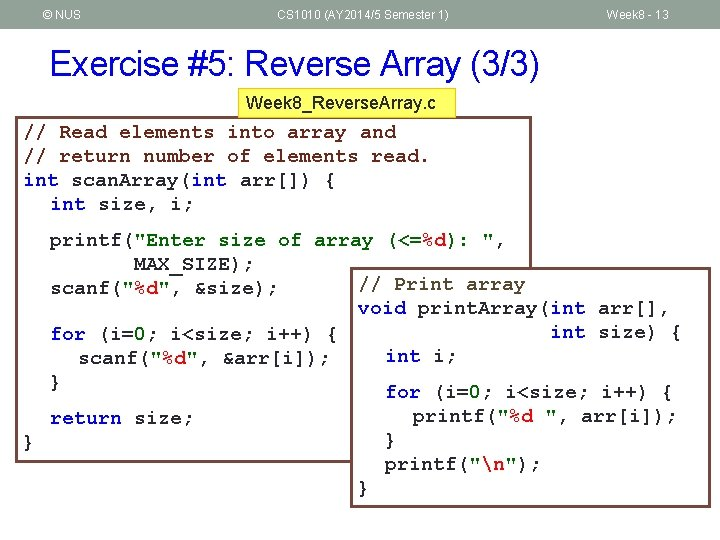© NUS CS 1010 (AY 2014/5 Semester 1) Week 8 - 13 Exercise #5: Reverse Array (3/3) Week 8_Reverse. Array. c // Read elements into array and // return number of elements read. int scan. Array(int arr[]) { int size, i; printf("Enter size of array (<=%d): ", MAX_SIZE); // Print array scanf("%d", &size); void print. Array(int arr[], int size) { for (i=0; i<size; i++) { int i; scanf("%d", &arr[i]); } for (i=0; i<size; i++) { printf("%d ", arr[i]); return size; } } printf("n"); }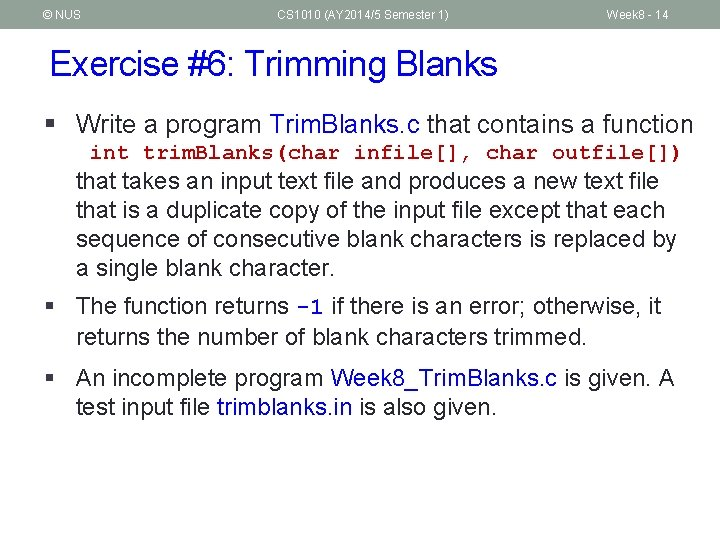© NUS CS 1010 (AY 2014/5 Semester 1) Week 8 - 14 Exercise #6: Trimming Blanks § Write a program Trim. Blanks. c that contains a function int trim. Blanks(char infile[], char outfile[]) that takes an input text file and produces a new text file that is a duplicate copy of the input file except that each sequence of consecutive blank characters is replaced by a single blank character. § The function returns -1 if there is an error; otherwise, it returns the number of blank characters trimmed. § An incomplete program Week 8_Trim. Blanks. c is given. A test input file trimblanks. in is also given.© NUS CS 1010 (AY 2014/5 Semester 1) Things-To-Do n Mid-Semester Test n n 11 October 2014, Saturday, 9: 30 am SR 1 and SR 3 Check CS 1010 “Term Tests” web page Continue to do practice exercises on Code. Crunch Week 8 - 15© NUS CS 1010 (AY 2014/5 Semester 1) End of File Week 8 - 16